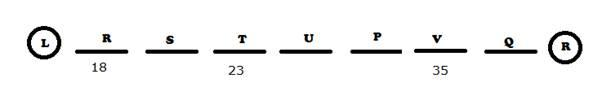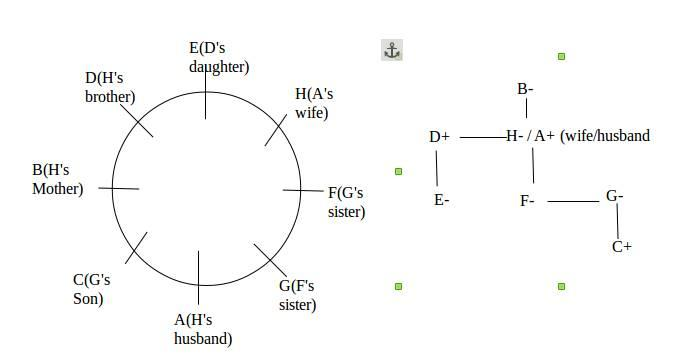## Seating Arrangement  For SBI PO Set – 34

( 1 – 5) Study the following information carefully and answer the given questions

Seven persons – P, Q, R, S, T, U and V – are seated in a straight line in the ascending order of their ages from left to right. V is older than U and S. V’s age is more than P but he is not the eldest. P is elder than U. The person whose age is 35 years, sitting second from the right end while the third Youngest person’s age is 23 years. T is younger than U but elder than S. R’s age is 18 years.

1) Who among the following is 35 years old?

a) P

b) U

c) Q

d) T

e) V

2) Who among the following is the eldest?

a) Q

b) V

c) P

d) U

e) T

3) Who among the following is elder than T but younger than P?

a) R

b) V

c) S

d) U

e) Q

4) Among the given persons, whose age may be 21 years old?

a) T

b) S

c) U

d) R

e) V

5) Who among the following persons are more than 23 but less than 35?

a) Only P

b) Only U

c) P and U

d) T and U

e) P and V1 e 2 a 3 d 4 b 5 c

( 6 -10) Study the following information carefully and answer the given questions

6) Who amongst the following is D’s daughter?

a) B

b) C

c) E

d) G

e) H

7) How many people sit between G and her uncle?

a) One

b) Two

c) Three

d) Four

e) More than four

8) Which of the following is true with respect to the given seating arrangement?

a) C is cousin of E.

b) H and H’s husband are immediate neighbours of each other.

c) No female is an immediate neighbour of C.

d) H sits third to the left of her daughter.

e) B is mother of H.

9) Four of the following five are alike in a certain way. Which is the one that does not belong to that group?

a) HC

b) BF

c) EA

d) DF

e) DG

10) What is the position of A with respect to his mother-in-law?

a) Immediate left

b) Third to the right

c) Third to the left

d) Second to the right

e) Fourth to the left6 e 7 c 8 e 9 d 10 d